# NCERT Solutions for Class 10 Science Chapter 12 ElectricityWritten by Team Trustudies
Updated at 2021-05-07

## NCERT solutions for class 10 Science Chapter 12 Electricity Exercise

Q1 )

A piece of wire of resistance R is cut into five equal parts. These parts are then connected in parallel. If the equivalent resistance of this combination is R', then the ratio R/R' is -

• (a) $$\frac{1}{25}$$
• (b) $$\frac{1}{5}$$
• (c) 5
• (d) 25

NCERT Solutions for Class 10 Science Chapter 12 Electricity

(d)25

Resistance of a piece of wire is proportional to its length. The given piece of wire has a resistance R. The wire is cut into five equal parts. Therefore, resistance of each part is
All the five parts are connected in parallel. Hence, equivalent resistance (R') is given asQ2 )

Which of the following terms does not represent electrical power in a circuit?

• (a)$$I^2R$$
• (b) $$IR^2$$
• (c)VI
• (d) $$V^2$$/R

NCERT Solutions for Class 10 Science Chapter 12 Electricity

(b)$$IR^2$$
Power of an appliance determines the rate at which electrical energy is delivered to it. Electrical power is given by the expression, P = VI … (i) According to Ohm's law, V = IR … (ii) where, V = Potential difference I = Current R = Resistance So, it can be written that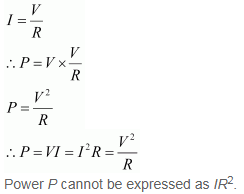Q3 )

An electric bulb is rated 220 V and 100 W. When it is operated on 110 V, the power consumed will be -

• (a) 100W
• (b) 75W
• (c)50W
• (d) 25W

NCERT Solutions for Class 10 Science Chapter 12 Electricity

(d)25W
Power of an appliance determines the rate at which electrical energy is delivered to it. Power of an appliance is given by the expression, P = VI = V2/R R =V2/P where, Power rating, P = 100 W Voltage, V = 220 V Resistance, R = 484 ohm The resistance of the bulb remains constant if the supply voltage is reduced to 110 V. If the bulb is operated on 110 V, then the energy consumed by it is given by the expression for power asQ4 )

Two conducting wires of the same material and of equal lengths and equal diameters are first connected in series and then parallel in a circuit across the same potential difference. The ratio of heat produced in series and parallel combinations would be -

• (a) 1:2
• (b) 2:1
• (c)1:4
• (d) 4:1

NCERT Solutions for Class 10 Science Chapter 12 Electricity

(c)1:4
Both the wires have the same resistance because they are made of the same material and have equal lengths and diameters. Let the resistance be R. For series combination, equivalent resistance Rs is Rs = R + R = 2R For parallel combination, equivalent resistance Rp is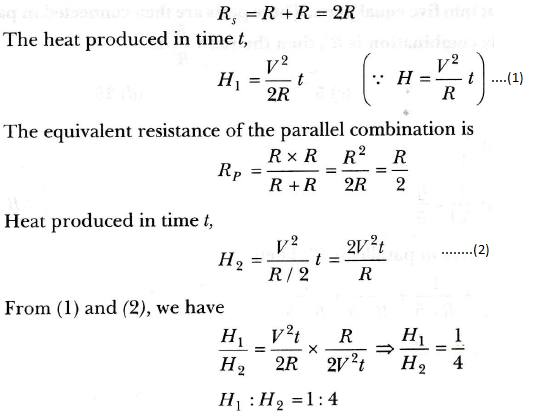Q5 ) How is a voltmeter connected in the circuit to measure the potential difference between two points ?

NCERT Solutions for Class 10 Science Chapter 12 Electricity

To measure the potential difference between two points, a voltmeter should be connected in parallel across these points.

Voltmeter is always connected in parallel to the element of any electrical circuit across which potential difference is to be measured.

Q6 ) A copper wire has diameter 0.5 mm and resistivity of $$1.6 × 10^-8 \Omega m.$$ What will be the length of this wire to make its resistance 10 $$\Omega$$? How much does the resistance change if the diameter is doubled?

NCERT Solutions for Class 10 Science Chapter 12 Electricity

Area of cross-section of the wire, A =$$\pi$$ (d/2) 2 Diameter= 0.5 mm = 0.0005 m Resistance, R = 10 $$\Omega$$ We know that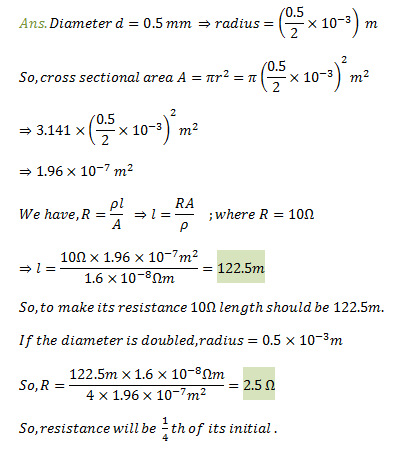Q7 )

The values of current I flowing in a given resistor for the corresponding values of potential difference V across the resistor are given below ?

 V(volts) 1.6 3.4 6.7 10.7 13.2 A(amperes) 0.5 1 2 3 4

Plot a graph between V and I and calculate the resistance of that resistor.

NCERT Solutions for Class 10 Science Chapter 12 Electricity

Answer The plot between voltage and current is called IV characteristic. The voltage is plotted on x-axis and current is plotted on y-axis. The values of the current for different values of the voltage are shown in the given table.

 V(volts) 1.6 3.4 6.7 10.7 13.2 A(amperes) 0.5 1 2 3 4

The V-I graph is shown below :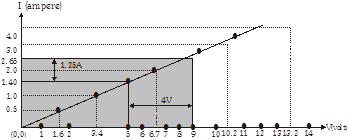the value of resistance R is $$R = \frac{V}{I} = \frac{4}{1.25} = 3.2 \Omega$$

Q8 )

When a 12 V battery is connected across an unknown resistor, there is a current of 2.5 mA in the circuit. Find the value of the resistance of the resistor.

NCERT Solutions for Class 10 Science Chapter 12 Electricity

Resistance (R) of a resistor is given by Ohm's law as,V= IR R=$$\frac{V}{I}$$ Where, Potential difference, V= 12 V Current in the circuit, I= 2.5 mA = 2.5 x 10-3 A $$R = \frac{12}{2.5} × 10^{-3} = 4.8 Kilo \Omega$$

Q9 ) A battery of 9 V is connected in series with resistors of 0.2 $$\Omega$$ , 0.3 $$\Omega$$, 0.4 $$\Omega$$, 0.5 $$\Omega$$ and 12 $$\Omega$$, respectively. How much current would flow through the 12 $$\Omega$$ resistor?

NCERT Solutions for Class 10 Science Chapter 12 Electricity

There is no current division occurring in a series circuit. Current flow through the component is the same, given by Ohm’s law as

V= IR
I= $$\frac{V}{R}$$

Where, R is the equivalent resistance of resistances 0.2 $$\Omega$$, 0.3 $$\Omega$$, 0.4 $$\Omega$$, 0.5 $$\Omega$$ and 12 $$\Omega$$. These are connected in series. Hence, the sum of the resistances will give the value of R.

R= 0.2 + 0.3 + 0.4 + 0.5 + 12 = 13.4 $$\Omega$$
Potential difference, V= 9 V
I= $$\frac{9}{13.4}$$ = 0.671 A

Therefore, the current that would flow through the 12 $$\Omega$$ resistor is 0.671 A.

Q10 ) How many 176 $$\Omega$$ resistors (in parallel) are required to carry 5 A on a 220 V line?

NCERT Solutions for Class 10 Science Chapter 12 Electricity

For x number of resistors of resistance 176 $$\Omega$$, the equivalent resistance of the resistors connected in parallel is given by Ohm's law as

V= IR R= $$\frac{V}{I}$$ Where, Supply voltage, V= 220 V Current, I = 5 A Equivalent resistance of the combination = R,given as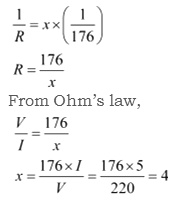Therefore, four resistors of 176 $$\Omega$$ are required to draw the given amount of current.

Q11 ) Show how you would connect three resistors, each of resistance 6 $$\Omega$$, so that the combination has a resistance of (i) 9 $$\Omega$$, (ii) 4 $$\Omega$$.

NCERT Solutions for Class 10 Science Chapter 12 Electricity

If we connect the resistors in series, then the equivalent resistance will be the sum of the resistors,

$$\Rightarrow$$ 6 $$\Omega$$ + 6 $$\Omega$$ + 6 $$\Omega$$ = 18 $$\Omega$$,

which is not desired. If we connect the resistors in parallel, then the equivalent resistance will be $$\frac{6}{2}$$ = 3 $$\Omega$$ is also not desired.

Hence, we should either connect the two resistors in series or parallel.

(a) Two resistor in parallel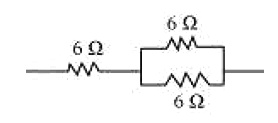Two 6 $$\Omega$$ resistors are connected in parallel. Their equivalent resistance will be
$$\frac{1}{(\frac{1}{6} + \frac{1}{6} )}= \frac{6}{2} = 3 \Omega .$$

The third 6 $$\Omega$$ resistor is in series with 3 $$\Omega$$. Hence, the equivalent resistance of the circuit is 6 $$\Omega$$+ 3 $$\Omega$$ = 9 $$\Omega$$.

(b) Two resistor in parallel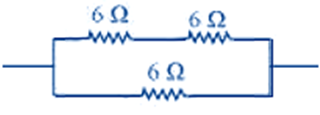Two 6 $$\Omega$$ resistors are in series. Their equivalent resistance will be the sum 6 + 6 = 12 $$\Omega$$.
The third 6 $$\Omega$$ resistor is in parallel with 12 $$\Omega$$. Hence, equivalent resistance will be

$$12 x \frac{6}{12} + 6 = 4 \Omega .$$

Therefore, the total resistance is 4$$\Omega$$

Q12 ) Several electric bulbs designed to be used on a 220 V electric supply line, are rated 10 W. How many lamps can be connected in parallel with each other across the two wires of 220 V line if the maximum allowable current is 5 A?

NCERT Solutions for Class 10 Science Chapter 12 Electricity

The resistance of the bulb can be calculated using the expression

$$P_1 = \frac{V_2}{R_1}$$
$$R_1 =\frac{ V_2}{P_1}$$.

Substituting the values, we get

R = $$\frac{220^2}{10} = 4840 \Omega$$
The resistance of x number of electric bulb is calculate as follows :

R =$$\frac{V}{I} = \frac{220}{5} = 44 \Omega$$

The resistance of each electric bulb is 4840 $$\Omega$$

The equivalent resistance of class bulb is given by :

$$\frac{1}{R} = \frac{1}{R1} + \frac{1}{R1}+ \frac{1}{R1} +....... up to x time$$

$$\frac{1}{R} = \frac{1}{R1}× x$$
x = $$\frac{R_1}{R} = \frac{4840}{44} = 110$$
Hence, 110 lamps can be connected in parallel.

Q13 ) A hot plate of an electric oven connected to a 220 V line has two resistance coils A and B, each of 24 $$\Omega$$resistances, which may be used separately, in series, or in parallel. What are the currents in the three cases?

NCERT Solutions for Class 10 Science Chapter 12 Electricity

Supply voltage, V= 220 V
Resistance of one coil, R= 24 $$\Omega$$

(i) Coils are used separately
According to Ohm's law,
$$V= I_1R_1$$
Where, $$I_1$$ is the current flowing through the coil

$$I_1 = \frac{V}{R_1} = \frac{220}{24} = 9.166 A$$

Therefore, 9.16 A current will flow through the coil when used separately.

(ii) Coils are connected in series

Total resistance, $$R_2$$ = 24 $$\Omega$$ + 24 $$\Omega$$ = 48 $$\Omega$$

According to Ohm's law,V = $$I_2R_2$$

Where, $$I_2$$ is the current flowing through the series circuit

$$I_2 = \frac{V}{R_2} = \frac{220}{48} = 4.58 A$$

Therefore, 4.58 A current will flow through the circuit when the coils are connected in series.

(iii) Coils are connected in parallel

Total resistance, R3 is given as $$= \frac{24}{2} = 12V$$

According to Ohm's law,
$$V= I_3R_3$$
Where,

I3 is the current flowing through the circuit $$I_3 = \frac{V}{R_3} = \frac{220}{12}= 18.33 A$$

Therefore, 18.33 A current will flow through the circuit when coils are connected in parallel.

Q14 ) Compare the power used in the 2 R resistor in each of the following circuits: (i) a 6 V battery in series with 1 R and 2 R resistors, and (ii) a 4 V battery in parallel with 12 R and 2 R resistors.

NCERT Solutions for Class 10 Science Chapter 12 Electricity

(i) Potential difference, V = 6 V

1 R and 2 R resistors are connected in series. Therefore, equivalent resistance of the circuit,

Resistance = 1 + 2 = 3 R
According to Ohm’s law, V = IR

Where, I is the current through the circuit

I= $$\frac{6}{3}$$ = 2 A

This current will flow through each component of the circuit because there is no division of current in series circuits. Hence, current flowing through the 2 $$\Omega$$ resistor is 2 A. Power is given by the expression,

P= (I)2R = (2)2 x 2 = 8 W

(ii) Potential difference, V = 4 V

12 R and 2 R resistors are connected in parallel. The voltage across each component of a parallel circuit remains the same. Hence, the voltage across 2 R resistor will be 4 V.

Power consumed by 2 $$\Omega$$ resistor is given by

$$P= \frac{V_2}{R} = \frac{42}{2} = 8 W$$

Therefore, the power used by 2 R resistor is 8 W.

Q15 ) Two lamps, one rated 100 W at 220 V, and the other 60 W at 220 V, are connected in parallel to electric mains supply. What current is drawn from the line if the supply voltage is 220 V?

NCERT Solutions for Class 10 Science Chapter 12 Electricity

Both the bulbs are connected in parallel. Therefore, potential difference across each of them will be 220 V, because no division of voltage occurs in a parallel circuit.

Current drawn by the bulb of rating 100 W is given by,Power = Voltage × Current

Current = $$\frac{Power}{Voltage} = \frac{60}{220} A$$

Hence, current drawn from the line
$$= \frac{100}{220} + \frac{60}{220} = 0.727 A$$

Q166 ) Which uses more energy, a 250 W TV set in 1 hr, or a 1200 W toaster in 10 minutes?

NCERT Solutions for Class 10 Science Chapter 12 Electricity

Energy consumed by an electrical appliance is given by the expression,H= Pt

Where, Power of the appliance = P
Time = t

Energy consumed by a TV set of power 250 W in 1 h
= 250 ×3600 = 9 ×105 J

Energy consumed by a toaster of power 1200 W in 10 minutes
= 1200 ×600

Energy consumed by a toaster of power 1200 W in 10 minutes
= 1200 ×600 = 7.2×105 J

Therefore, the energy consumed by a 250 W TV set in 1 h is more than the energy consumed by a toaster of power 1200 W in 10 minutes.

Q17 ) An electric heater of resistance 8$$\Omega$$ draws 15 A from the service mains 2 hours. Calculate the rate at which heat is developed in the heater.

NCERT Solutions for Class 10 Science Chapter 12 Electricity

Rate of heat produced by a device is given by the expression for power as, P=$$I_2R$$

Where, Resistance of the electric heater, R= 8 $$\Omega$$

Current drawn, I = 15 A
P= (15)2 x 8 = 1800 J/s

Therefore, heat is produced by the heater at the rate of 1800 J/s.

Q18 )

Explain the following

1. Why is the tungsten used almost exclusively for filament of electric lamps?
2. Why are the conductors of electric heating devices, such as bread-toasters and electric irons, made of an alloy rather than a pure metal?
3. Why is the series arrangement not used for domestic circuits?
4. How does the resistance of a wire vary with its area of cross-section?
5. Why are copper and aluminium wires usually employed for electricity transmission?

NCERT Solutions for Class 10 Science Chapter 12 Electricity

1. The melting point and of Tungsten is an alloy which has very high melting point and very high resistivity so does not burn easily at a high temperature.
2. The conductors of electric heating devices such as bread toasters and electric irons are made of alloy because resistivity of an alloy is more than that of metals which produces large amount of heat.
3. In series circuits voltage is divided. Each component of a series circuit receives a small voltage so the amount of current decreases and the device becomes hot and does not work properly. Hence, series arrangement is not used in domestic circuits.
4. Resistance (R) of a wire is inversely proportional to its area of cross-section (A), i.e. when area of cross section increases the resistance decreases or vice versa.
5. Copper and aluminium are good conductors of electricity also they have low resistivity. So they are usually used for electricity transmission.

21. An electric iron of resistance 20Ω takes a current of 5 A. Calculate the heat developed in 30 s.

NCERT Solutions for Class 10 Science Chapter 12 Electricity

Given, Electric current (I) = 5A, Resistance (R) = 20Ω, Time (t) = 30s
Heat developed, H = $$I^2 R t$$ = 25 x 20 x 30 = 15,000 J \)

We have, H = $$1.5 x 10^4 J$$

## NCERT solutions for class 10 Science Chapter 12 Electricity Intext Exercise

Q1 ) What does an electric circuit mean?

NCERT Solutions for Class 10 Science Chapter 12 Electricity

A continuous path along which an electric current flows is called an electric circuit.

Q2 ) Define the unit of current.

NCERT Solutions for Class 10 Science Chapter 12 Electricity

SI Unit of current is ampere(A). If one coulomb of electric charge flows through any section of a conductor in one second, then the current through it is said to be one ampere.

$$I= \frac{Q}t$$ or $$1 A = 1 C s^{-1}$$

Q3 ) Calculate the number of electrons constituting one coulomb of charge.

NCERT Solutions for Class 10 Science Chapter 12 Electricity

Charge on one electron, e = $$1.6$$x $$10^{-19}C$$
Total charge, Q = 1 C

Number of electrons, n = $$\frac{Q}e$$

n= $$\frac{1C}{1.6*10^{-19}}$$= 6.25 x $$10^{18}$$

Q4 ) Name a device that helps to maintain a potential difference across a conductor.

NCERT Solutions for Class 10 Science Chapter 12 Electricity

A battery or a cell.

Q5 ) What is meant by saying that the potential difference between two points is 1V?

NCERT Solutions for Class 10 Science Chapter 12 Electricity

If 1 joule of work is done in moving 1 coulomb of electric charge between two points then, the potential difference between two points is said to be 1 volt .

Q6 ) How much energy is given to each coulomb of charge passing through a 6 V battery?

NCERT Solutions for Class 10 Science Chapter 12 Electricity

Charge Q = 1C, Potential difference, V = 6V
Energy given by battery = charge x potential difference

or W = QxV

W= 1C X 6V = 6J.

Q7 ) On what factors does the resistance of a conductor depend ?

NCERT Solutions for Class 10 Science Chapter 12 Electricity

The resistance of a conductor depends upon:
(i)its length.

(ii)area of cross-section of conductor.

(iii)the nature of its material of conductor.

Q8 ) Will current flow more easily through a thick wire or a thin wire of the same material, when connected to the same source ? Why ?

NCERT Solutions for Class 10 Science Chapter 12 Electricity

As we know that resistance is indirectly proportional to the area of cross section, thus current flows easily through a thick wire compared to a thin wire of the same material.

Q9 ) Let the resistance of an electrical component remain constant while the potential difference across the two ends of the component decreases to half of its former value. What change will occur in the current through it?

NCERT Solutions for Class 10 Science Chapter 12 Electricity

According to ohm’s law we have V is directly proportion to I.
So when potential difference between two ends of the component is halved, the current through the component also decreases to half of its initial value.

Q10 ) Why are coils of electric toasters and electric irons are made of an-alloy rather than a pure metal ?

NCERT Solutions for Class 10 Science Chapter 12 Electricity

The coils of electric toasters and electric irons are made of an alloy rather than a pure metal because the resistivity of an alloy is much higher than that of a pure metal, and also at high temperatures, the alloys do not melt easily i.e, the melting point of alloys is higher than that of pure metals to retain more heat without melting.

Q11 ) Use the data in Table 12.2 (in NCERT Book on Page No. 207) to answer the following :
(i) Which among iron and mercury is a better conductor ?

(ii) Which material is the best conductor ?

NCERT Solutions for Class 10 Science Chapter 12 Electricity

(i) Resistivity of iron = $$10.0$$x $$10^{-8} \Omega m$$
Resistivity of mercury =$$94.0$$ x $$10^{-8} \Omega m$$.

Therefore, iron is a better conductor because it has lower resistivity than mercury.

(ii) silver has the lowest resistivity ($$1.60$$ x $$10^{-8} \Omega m$$), therefore silver is the best conductor.

Q12 ) Draw a schematic diagram of a circuit consisting of a battery of three cells of 2 V each, a 5 $$\Omega$$ resistor, an 8 $$\Omega$$ resistor, and a 12$$\Omega$$ resistor, and a plug key, all connected in series.

NCERT Solutions for Class 10 Science Chapter 12 Electricity

The required circuit diagram is:Q13 ) Redraw the circuit of Question 1, putting in an ammeter to measure the current through the resistors and a voltmeter to measure the potential difference across the 12 $$\Omega$$ resistor. What would be the readings in the ammeter and the voltmeter?

NCERT Solutions for Class 10 Science Chapter 12 Electricity

Net resistance in the circuit(R) = Sum of the resistances of all resistors
R= 5 $$\Omega$$+ 8 $$\Omega$$ + 12 $$\Omega$$ = 25$$\Omega$$

We have; $$R=\frac{V}I$$

$$25 \Omega =\frac{6V}I$$

or $$I=\frac{6V}{25 \Omega }=0.24A$$

Electric current remains the same through all resistors because resistances are connected in series.

Here we have,

Electric current, $$I$$ = 0.24A

Resistance, R = 12$$\Omega$$

So, potential difference, V through the resistor of 12 $$\Omega$$ = $$I$$ x R

Or, V = 0.24A x 12 $$\Omega$$ = 2.88 V

Thus, reading of ammeter = 0.24A

Reading of voltmeter through resistor of 12 $$\Omega$$ = 2.88V

And the circuit can be formed as:Q14 ) Judge the equivalent resistance when the following are connected in parallel –
(a) 1 $$\Omega$$ and 106$$\Omega$$,

(b) 1$$\Omega$$ and 103$$\Omega$$, and 106$$\Omega$$.

NCERT Solutions for Class 10 Science Chapter 12 Electricity

When the resistances are connected in parallel, the equivalent resistance is smaller than the smallest individual resistance.so here we have the smallest resistance as 1 $$\Omega$$ so,

(i) Equivalent resistance < 1$$\Omega$$.

(ii) Equivalent resistance < 1$$\Omega$$.

Q15 ) An electric lamp of 100 $$\Omega$$ , a toaster of resistance 50 $$\Omega$$, and a water filter of resistance 500 $$\Omega$$ are connected in parallel to a 220 V source. What is the resistance of an electric iron connected to the same source that takes as much current as all three appliances, and what is the current through it ?

NCERT Solutions for Class 10 Science Chapter 12 Electricity

Resistance of electric lamp, $$R_1 = 100 \Omega$$
Resistance of toaster, $$R_2 = 50 \Omega$$

Resistance of water filter, $$R_3 = 500 \Omega$$

Equivalent resistance R of the three appliances connected in parallel, is:

$$\frac{1}R=\frac{1}{R_1} +\frac{1}{R_2}+\frac{1}{R_3}$$

$$\frac{1}R= \frac{1}{100} +\frac{1}{50}+\frac{1}{500}=\frac{16}{500}$$

$$R=\frac{500}{16} \Omega =31.25 \Omega$$

Resistance of electric iron = Equivalent resistance of the three appliances connected in parallel = 31.25 $$\Omega$$

Applied voltage, V = 220 V

So,current$$I=\frac{v}R =\frac{220}{31.25}=7.04A$$

Q16 ) What are the advantages of connecting electrical devices in parallel with the battery instead of connecting them in series ?

NCERT Solutions for Class 10 Science Chapter 12 Electricity

Advantages of connecting electrical devices in parallel are :

(i)In parallel circuits, if an electrical appliance stops working due to some defect, then all other appliances keep working normally and also each electrical appliance has its own switch due to which it can be turned on turned off independently, without affecting other appliances.

(ii)In parallel circuits,the same voltage(220V) from the power supply line would be supplied to each electrical appliance.

(iii)In the parallel connection of electrical appliances, the overall resistance of the household circuit is reduced due to which the current from the power supply is high.

Q17 ) How can three resistors of resistances 2$$\Omega$$, 3$$\Omega$$ , and 6$$\Omega$$ be connected to give a total resistance of (a) 4$$\Omega$$, (b) 1$$\Omega$$?

NCERT Solutions for Class 10 Science Chapter 12 Electricity

(i) We can get a total resistance of 4$$\Omega$$ by connecting the 2$$\Omega$$ resistance in series with the parallel combination of 3$$\Omega$$ and 6$$\Omega$$.
So, $$R=R_1+\frac{R_2R_3}{R_2+R_3}= 2+\frac{6×3}{6+3}=4\Omega$$

(ii) We can obtain a total resistance of 1$$\Omega$$ by connecting resistors of 2$$\Omega$$, 3$$\Omega$$ and 6$$\Omega$$ in parallel.

$$\frac{1}R=\frac{1}{R_1} +\frac{1}{R_2}+\frac{1}{R_3}=\frac{1}{2} +\frac{1}{3}+\frac{1}{6}$$

$$\frac{1}R=1 \Omega$$

or R=1 $$\Omega$$

Q18 ) What is (i) the highest, (ii) the lowest total resistance that can be secured by combinations of four coils of resistance 4$$\Omega$$ , 8$$\Omega$$, 12$$\Omega$$, 24$$\Omega$$ ?

NCERT Solutions for Class 10 Science Chapter 12 Electricity

(i) we can get highest resistance by connecting the four coils in series.
Then, R = 4$$\Omega$$ + 8$$\Omega$$ + 12$$\Omega$$ + 24$$\Omega$$ = 48$$\Omega$$

(ii)We can get lowest resistance by connecting the four coils in parallel.

$$\frac{1}R=\frac{1}4 +\frac{1}8+\frac{1}{12} =\frac{12}{24}=\frac{1}2$$

we get, R=2$$\Omega$$

Q19 ) Why does the cord of an electric heater not glow while the heating element does ?

NCERT Solutions for Class 10 Science Chapter 12 Electricity

The resistance of the heating element is very high compared to the cord of an electric heater. Since,Heat generated in a circuit is given by $$I^2Rt$$ so heat produced is directly proportional to the resistance, thus element of electric heater glows because of production of more heat due to high resistance but the cord of the electric heater made of copper does not glow because negligible heat is produced in it by passing current because of its extremely low resistance.

Q20 ) Compute the heat generated while transferring 96000 coulomb of charge in one hour through a potential difference of 50 V.

NCERT Solutions for Class 10 Science Chapter 12 Electricity

Given, Potential difference (V) = 50V,
Charge (Q) = 96000 coulomb

Time, t = 1 hour = 60 X 60 s = 3600 s

Heat generated, H = VQ = 50Vx 96,000 C = 48,00,000 J = 4.8 x $$10^6$$ J

Q22 ) What determines the rate at which energy is delivered by a current ?

NCERT Solutions for Class 10 Science Chapter 12 Electricity

Rate at which energy is delivered by a current is the power of electric appliance as electric power is the rate of consumption of electric energy in any electrical appliance.

Q23 ) An electric motor takes 5 A from a 220 V line. Determine the power of the motor and the energy consumed in 2 h.

NCERT Solutions for Class 10 Science Chapter 12 Electricity

Given, Electric current (I) = 5A,
Potential difference (V) = 220V,

Time (t) = 2h = 2 x 60 x 60 s = 7200 s

We know,Power (P) = V I = 220 x 5

P= 1100 W

Energy consumed by the electric appliance = P × t=1100 W × 7200 s

Energy consumed= 7.92 × $$10^6$$ J

##### FAQs Related to NCERT Solutions for Class 10 Science Chapter 12 Electricity
There are total 41 questions present in ncert solutions for class 10 science chapter 12 electricity
There are total 2 long question/answers in ncert solutions for class 10 science chapter 12 electricity
There are total 2 exercise present in ncert solutions for class 10 science chapter 12 electricity